# NCERT Solutions for Class 10 Chemistry Chapter 1 - Chemical Reactions and Equations

Find out how to create a balanced chemical equation and learn about chemical reactions with CBSE Class 10 Chemistry learning resources. On TopperLearning, our experts support you to understand chemistry with CBSE Class 10 Chemistry notes such as CBSE Class 10 Chemistry notes, MCQs and NCERT solutions as per the latest syllabus.

Discover the nomenclature of organic compounds, properties of hydrocarbons and a lot more through our CBSE Class 10 Chemistry Carbon and its compounds worksheets. Learn to identify elements based on their properties using our CBSE Class 10 Chemistry Periodic Classification of Elements notes.

For practicing questions and answers, you can check out our CBSE Class 10 Chemistry Chapter-1 solutions for NCERT textbook questions. Similarly, you can move ahead by practicing CBSE Class 10 Chemistry Chapter-2 questions or reviewing CBSE Class 10 Chemistry Chapter-2 notes.

In the CBSE Class 10 Science Syllabus 2019-2020, you will find 25 marks allocated to Chemical Substances – Nature and Behaviour in Unit-I. You can easily score those marks by practicing questions from our CBSE Class 10 Chemistry sample papers with answers.

Learning the fundamentals of Chemistry in CBSE Class 10 is now easy. TopperLearning presents study materials for CBSE Class 10 Chemistry which will help you to achieve a good score in your examination. Here, we believe in making students understand the basic principles and terminology. So, we have created highly interactive videos wherein all the concepts are explained in detail. Our study materials consist of video lessons, revision notes, question banks, sample papers and past year question papers which give you deeper insight on topics in Chemistry.

All our study materials are created by subject experts who have experience in teaching. Chemistry is an important subject and is a crucial subject in Science, so learning the fundamentals is important. While creating the study materials, we personally take care that they are in accordance with the latest CBSE syllabus and revised according to the changes prescribed. Apart from our study materials, students can also learn from our NCERT and RD Sharma textbook solutions which are available free of cost. Our comprehensive set of study materials acts as a perfect guide when doing homework and preparing for the examination.

Page / Exercise

## Chapter 1 - Chemical Reactions and Equations Exercise 10

Solution 1
(i) The substance 'X' is calcium oxide (lime). Its chemical formula is CaO.
(ii) Calcium oxide reacts vigorously with water to form calcium hydroxide (slaked lime).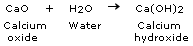Concept insight: The key to this answer is white washing. Recall the substance used for white washing and answer accordingly.
Solution 2

Water (H2O) contains two parts of hydrogen and one part of oxygen. Therefore, the amount of hydrogen and oxygen produced during electrolysis of water is in a 2:1 ratio. During electrolysis, since hydrogen goes to one test tube and oxygen gas goes to another hence, the amount of gas collected in one of the test tubes is double the amount collected in the other.  The gas collected in double the amount is hydrogen and the other gas is oxygen.

Concept insight: The key to this answer is the electrical decomposition reaction of water.

## Chapter 1 - Chemical Reactions and Equations Exercise 13

Solution 1
When an iron nail is placed in a copper sulphate solution, iron displaces copper from the blue colored copper sulphate solution forming light green colored ferrous sulphate solution and copper metal.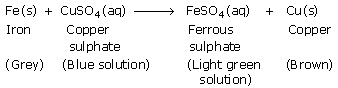Concept insight:  The key to this answer is that a displacement reaction takes place when iron nails are dipped in copper sulphate solution. Recall that iron is a more reactive metal than copper.
Solution 2

A double displacement reaction between the reactants lead nitrate and potassium iodide gives new substances: a yellow ppt. of lead iodide and potassium nitrate.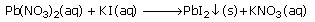In this reaction, lead nitrate and potassium iodide exchange ions to form two new compounds lead iodide and potassium nitrate. Hence, it is a double displacement reaction.

Concept insight:  Recall that two different atoms or groups of atoms (ions) are exchanged in double displacement reactions.

Solution 3

(i) Sodium (Na) is oxidised to sodium oxide as it gains oxygen and oxygen (O2) gets reduced.

(ii) Copper oxide (CuO) is reduced to copper (Cu) while hydrogen (H2) gets oxidised to water (H2O).

Concept insight:  Recall that oxidation reaction is gain of oxygen and reduction reaction is release of oxygen.

## Chapter 1 - Chemical Reactions and Equations Exercise 14

Solution 1

(i) (a) and (b)

Concept insight: Recall that oxidation reaction is gain of oxygen and reduction reaction is release of oxygen.

Solution 2
(d) displacement reaction

Concept insight: The key to this answer is to recall all the type of chemical reactions and match with the definitions. It cannot be a combination or decomposition reaction since combination reaction involves one product and decomposition reaction involves one reactant. Now, match it with displacement and double displacement reaction and see where it fits - whether two displacements are taking place or one. If one displacement is taking place, it is displacement reaction and if two displacements are taking place, it is double displacement reaction.

## Chapter 1 - Chemical Reactions and Equations Exercise 15

Solution 1
(a) Hydrogen gas and iron chloride are produced.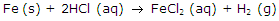Concept insight: The key to this answer is to remember that metals react with hydrochloric acid to form respective metal chlorides and liberate hydrogen gas.
Solution 2

A chemical equation is balanced when the numbers of atoms of each type involved in a chemical reaction are same on both the reactant and product sides of the equation.

The chemical equations must always be balanced to satisfy the law of conservation of mass which states that "Mass can neither be created nor destroyed in a chemical reaction." This means that the total mass of the elements present in the products of a chemical reaction has to be equal to the total mass of the elements present in the reactants. Hence, the number of atoms of each element in the products must be equal to the number of atoms of these elements in the reactants.

Concept insight: Rememeber that a chemical equation should be balanced to follow the Law of conservation of mass.

Solution 3
(a) 3H2 + N22NH3
(b) 2H2S + 3O22H2O + 2SO2
(c) 3BaCl2 + Al2(SO4)32AlCl3 + 3BaSO4
(d) 2K + 2H22KOH + H2
Concept insight: First convert the word equation into skeletal equation and then balance the different atoms on both sides of the equation.
Solution 4
(a) 2HNO3 + Ca(OH)2Ca(NO3)2 + 2H2O
(b) 2NaOH + H2SO4Na2SO4 + 2H2O
(c) NaCl + AgNO3AgCl + NaNO3
(d) BaCl2 + H2SO4BaSO4 + 2HCl
Concept insight: First write all the different types of atoms on both the reactant and product side. Balance them one by one

Solution 5
(a) Ca(OH)2 + CO2CaCO3 + H2O
(b) Zn + 2AgNO3Zn(NO3)2 + 2Ag
(c) 2Al + 3CuCl22AlCl3 + 3Cu
(d) BaCl2 + K2SO4BaSO4 + 2KCl

Concept insight: First convert the word equation into skeletal equation and then balance the different atoms on both sides of the equation.
Solution 6

(a) 2KBr (aq) + Bal22Kl (aq) + BaBr2 (s), Double displacement reaction and precipitation reaction

(b) ZnCO3 (s)ZnO (s) + CO2 (g), Decomposition reaction

(c) H2 (g) + Cl2 (g)2HCl(g), Combination reaction

(d) Mg(s) + 2HCl(aq)MgCl2 (s) + H2 (g), Displacement reaction

Concept insight: First convert the word equation into skeletal equation and then balance the different atoms on both sides of the equation. Write the states of all the reactants and products in bracketts. Then identify the type of reaction by recalling all the type of chemical reactions and match with the reactions one by one. A combination reaction involves one product and decomposition reaction involves one reactant. For identifying whether it is displacement reaction or not, see whether two displacements are taking place or one. If one it is displacement reaction and if two displacements are taking place, it is double displacement reaction.

Solution 7

Reactions in which heat is released along with the formation of products are called exothermic reactions. Example of exothermic reaction:

Burning of natural gas: CH4(g) + 2O2 (g)CO2 (g) + 2H2O (g) + Heat

Reactions in which energy is absorbed are known as endothermic reactions. Example of endothermic reaction:

2AgBr(s)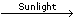2Ag(s) + Br2(g)

Concept insight: For this answer, you should remember that in one reaction, energy is released and in the other, energy is absorbed. Exo means out of or outside so exothermic means that energy is released. Endo means inside so endothermic means that energy is absorbed.

Solution 8
Food that we eat includes carbohydrates, proteins, vitamins etc. During digestion, carbohydrates are broken down into simpler substances called glucose. Glucose combines with oxygen in the cells of our body to form carbon dioxide and water along with energy. This reaction is called respiration. Since energy is released during this process, respiration is an exothermic reaction.Concept insight: For answering this question, you should know what exothermic reactions are and then relate it to respiration.

Solution 9

In a decomposition reaction, a single substance decomposes to give two or more substances. Whereas in a combination reaction two or more substances combine to form a new single substance. And hence, decomposition reactions are opposite of combination reactions.

Decomposition reaction: AB + EnergyA + B

Example:

CaCO3(s)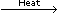CaO(s) + CO2(g)

Combination reaction: A + BAB + Energy

Example: Burning of coal : C(s) + O2(g)CO2(g)
Concept insight: For answering this question, you should know what combination and decomposition reactions are and then reason whether they are opposite of each other or not.

## Chapter 1 - Chemical Reactions and Equations Exercise 16

Solution 1

Heat              CaCO3(s)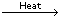CaO(s) + CO2(g)

Light              2AgBr(s)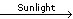2Ag(s) + Br2(g)

Electricity       2H2O (l)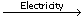2H2 (g) + O2 (g)

Concept insight: For answering this question, you have to write a complete balanced chemical reaction for all the three types of reactions.

Solution 2

A displacementreaction is a chemical reaction in which a more reactive element displaces a less reactive element from its salt solution.

Example:
Fe + CuSO4FeSO4 + Cu
In this reaction, one displacement is taking place. Fe is displacing Cu.

Double displacement reaction is a chemical reaction in which there is an exchange of ions between the reactants to give new substances. There are two displacements taking place in a double displacement reaction.

Example:
3 BaCl2 + Al2(SO4)32 AlCl3 + 3 BaSO4
In this reaction, two displacements are taking place. Ba is displacing Al and Al is displacing Ba.

Concept insight: For answering this question, you should know what displacement and double displacement reaction are. If one displacement is taking place, it is displacement reaction and if two displacements are taking place, it is double displacement reaction.

Solution 3
2AgNO3 (aq) + Cu(s)Cu(NO3)2 + 2Ag(s)

Concept insight: For answering this question, write the chemical reaction between silver nitrate solution and copper metal.
Solution 4

Any reaction that produces an insoluble solid (precipitate) can be called a precipitation reaction.

These insoluble salts separate out from the solution and settle down as precipitate.

For example: When aqueous sodium sulphate solution and aqueous barium chloride are reacted, aqueous solution of sodium chloride and white precipitate of barium sulphate are formed.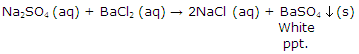Concept insight: For answering this question, you should recall what precipitate is. Write the definition of precipitation reaction. Then, state an example by writing the balanced chemical equation.

Solution 5

(a) Oxidation: It is the gain of oxygen by a substance in a reaction.

Example 1: When magnesium is burned in air, magnesium oxide is formed.

2Mg + O22MgO

Here, magnesium is oxidized to magnesium oxide. It has gained oxygen.

Example 2: When copper oxide is heated with hydrogen, copper metal and water are formed.

CuO + H2Cu + H2O

Here, H2 is getting oxidised to H2O. It has gained oxygen.

(b) Reduction: It is the loss of oxygen by a substance in a reaction.

Example 1: When zinc oxide is heated with carbon, zinc metal and carbon monoxide are formed.

ZnO + CZn + CO

Here, ZnO is getting reduced to Zn. It has lost oxygen.

Example 2: When copper oxide is heated with hydrogen, copper metal and water are formed.

CuO + H2Cu + H2O

Here, CuO is getting reduced to Cu. It has lost oxygen.

Concept insight: In this reaction, remember that you have to write the definition of oxidation and reduction in terms of oxygen atoms. Also, remember that you have to state two examples.
Solution 6
The shiny brown coloured element 'X' is copper and the black coloured compound is copper oxide (CuO) .The chemical reaction is:

2Cu + O2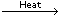2CuO

Concept insight: In this reaction, recall the element which is shiny brown in colour. When it will be heated in air, it will form its oxide. So, which oxide is black in colour?

Solution 7

We apply paint on iron articles to avoid their rusting. When a coat of paint is applied to the surface of an iron article, it stops the contact of air and moisture with the iron metal and hence no rusting takes place.

Concept insight: In this reaction, it is to think that what will happen to iron articles if we do not apply paint on them. Iron will react with air and moisture and corrode. Therefore, to prevent corrosion of iron articles we have to apply paint on them.

Solution 8
When fats and oils are kept for a long time, they get oxidized by aerial oxidation and become rancid and their smell and taste change. Food items containing oil and fat are flushed with nitrogen to prevent rancidity of oil and fat as nitrogen is an inert gas and prevent the oxidation of oil and fats.

Concept insight: In this reaction, it is to think that what the properties of nitrogen gas are. It is inert. If it is inert, it will not react with fats and oils and can be used for preventing rancidity.

Solution 9

(a) Corrosion: Corrosion is the process of eating away of metals by the attack of substances such as moisture, acids, etc. Example - the black coating on silver and the green coating on copper are other examples of corrosion.

(b) Rancidity: When food items containing fats and oils are kept for a long time, they get oxidized and their smell and taste change. This process is known as rancidity.

Example: Butter when kept in open for long time, tastes and smells bad because of rancidity.

Concept insight: For this answer, write complete definitions of corrosion and rancidity. Also, don't forget to write one example of each.

## Chapter 1 - Chemical Reactions and Equations Exercise 6

Solution 1

When magnesium ribbon is stored, it reacts with oxygen of air to form a layer of magnesium oxide. This layer of magnesium oxide is quite stable and prevents further reaction of magnesium with oxygen. Hence, a magnesium ribbon should be cleaned before burning in air to remove this layer of magnesium oxide.

Concept insight: Always remember that magnesium being a reactive metal will react with oxygen if kept in open. So, it has to be cleaned before it is burned in air.

Solution 2
(i) H2 + Cl22HCl
(ii) 3BaCl2 + Al2 (SO4)33BaSO4 + 2AlCl3
(iii) 2Na + 2H22NaOH + H2
Concept insight: First convert the word equation into skeletal equation and then balance the different atoms on both sides of the equation.

Solution 3
(i) BaCl2 (aq) + Na2SO4 (aq)BaSO4 (S) + 2NaCl (aq)
(ii) NaOH (aq) + HCl (aq)NaCl (aq) + H2O (l)
Concept insight: First convert the word equation into skeletal equation and then balance the different atoms on both the sides of the equation. Also, do not forget to write the symbols for different states.

## Why choose TopperLearning’s CBSE Class 10 study materials?

On TopperLearning, you can find chapter-wise learning resources for all 5 chapters and 21 topics covered in the CBSE Class 10 Chemistry Syllabus. To make chemistry learning simpler, we have given sample papers and solutions, CBSE Class 10 Chemistry Previous Year Papers and Solutions, chapter notes, videos and lots more.

We make your learning process easier by providing all the essential CBSE Class 10 Chemistry learning materials such as NCERT solutions, RD Sharma notes etc. along with engaging learning videos. Also, solving doubts effectively is simple with our student forums and doubt clarification services. Becoming a CBSE topper is now possible by using the intuitive TopperLearning student dashboard to prepare for your CBSE Class 10 exams.

CONTACT :
1800-212-785810:00 AM to 7:00 PM IST all days .
SUBSCRIBE TO TOPPER :
Explore